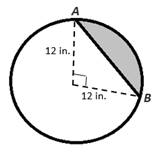Chapter 8.CT, Problem 15CT### Elementary Geometry for College St...

6th Edition
Daniel C. Alexander + 1 other
ISBN: 9781285195698

#### Solutions

Chapter
Section### Elementary Geometry for College St...

6th Edition
Daniel C. Alexander + 1 other
ISBN: 9781285195698
Textbook Problem
1 views

# Find the exact area of the shaded segment.                                 _To determine

To find:

The exact area of the shaded segment.

Explanation

Given:

The figure below.

Theorem:

1. In a circle of radius length r, the area A of a sector whose arc has degree measure m is given by A=m360πr2.

2. The area A of an isosceles triangle whose length of the equal sides r is given by A=12r2.

From the figure given, r=12 in., and m=90.

Area of the segment = Area of the sector – Area of the triangle

A=m360πr2-12

### Still sussing out bartleby?

Check out a sample textbook solution.

See a sample solution

#### The Solution to Your Study Problems

Bartleby provides explanations to thousands of textbook problems written by our experts, many with advanced degrees!

Get Started

#### Convert the expressions in Exercises 6584 to power form. 35x2

Finite Mathematics and Applied Calculus (MindTap Course List)

#### In Exercises 73-80, find the indicated limits, if they exist. 75. limx3x3+x2+1x3+1

Applied Calculus for the Managerial, Life, and Social Sciences: A Brief Approach

#### Multiply. (2+4x)(3x)

Trigonometry (MindTap Course List)

#### True or False: If f(c) exists and f has a local minimum at c, then f(c) = 0.

Study Guide for Stewart's Single Variable Calculus: Early Transcendentals, 8th